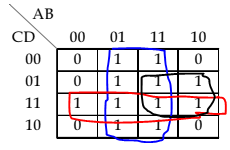# UGC NET COMPUTER SCIENCE SOLVED PAPERS 2017-19 - UGC NET Computer Science November 2017 Paper 2

>>>>>>>>UGC NET Computer Science November 2017 Paper 2

is:

• A

(A+C).D+B• B

(A+B).C+D• C

(A+D).C+B• D

(A+C).B+D• Option : A
• Explanation :
To find the boolean function here we will use K-map Select neighboring Minterms as max as possible in powers of 2 ie 2,4,8 and so on.Here we have selected group of minterms: First we will take 8 minterms group which will give us B. Now take 4 minterms group it will give us CD. Another 4 minterms group will give us AD. We have the expression B + CD + AD. which can be reduced as B + D ( A + C )
So, option (A) is correct.

• A

7353• B

1353• C

5651• D

5657• Option : B
• Explanation :
First we will make pair of 3 bits from LSB : ie- 1 011 101 011 now convert these bits into decimal and that will be 1 - 1 011 - 3 101 - 5 011 - 3 so the answer will be 1353.
So, option (B) is correct.

• A

Only (I) and (II)• B

Only (II) and (III)• C

Only (III) and (IV)• D

None of the above• Option : A
• Explanation :
We know that ¬(P ↔ Q) = (¬P ↔ Q) = (P ↔ ¬Q) = ¬(¬P ↔ ¬Q) Only statement (I) and (II) are correct.
So, option (A) is correct.

• A

*(ptrdata++)• B

(*ptrdata)++• C

*(ptrdata)++• D

Depends on compiler• Option : A
• Explanation :
Here ++ (unary operator) have high precedence than * (unary operator). So ++ will be evaluated first then * will be in action. So A is the correct option. So, option (A) is correct.

• A

Unary Operator• B

Logical not• C

Array element access• D

addressof• Option : C
• Explanation :
Array element access has left to right associativity in C++.
So, option (C) is correct.